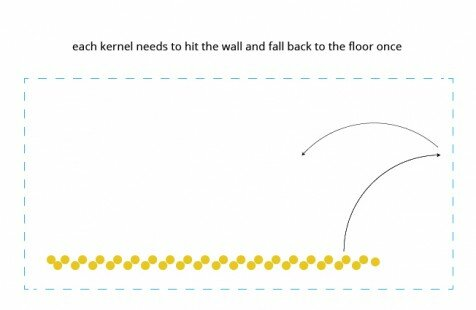# Popcorn fail

This week I watched all the videos from chapters and initially tried to work with Javascript.
It was maybe too much to take at once – the math concepts and a new language so I went back to processing and Java for now, until I get used to working with the browser and js.

My goal was to create a movement that emulates popcorn, as you can see in this sketchI managed to get one kernel bouncing but I couldn’t get it to do it only once.
Also, when I tried to make an array of kernels, I keep getting a Null pointer exception error.

Here is my buggy code for now:

Corn[] c ;
int count = 100;

void setup() {
size(700,700);
c= new Corn;

}
void draw() {
background(255);

fill(0);
rect(0,350,700,350);

for (int i = 0; i < 10; i++) {
c[i].display();
c[i].checkEdges();

}}

class Corn{
PVector location;
PVector velocity;
PVector acceleration;
float gravity = 0.48;
float vy = 0;
float bounce = -1;

Corn(){
location = new PVector(random(100,600),(height/2));
velocity = new PVector(1,1);
acceleration = new PVector(random(0,0.02),random(0.1,0.2));
}
// void update() {
// velocity.sub(acceleration);
// }
void display() {
noStroke();
fill(#FFCC00);
ellipse(location.x, location.y, 10, 10);
translate(width/2, height/2);

}
void checkEdges() {
vy += gravity;
location.y+=vy;
if(location.y > height – 15)
{
vy *= bounce;
}
else if (location.y < 0) {
location.y = height;
}
}

}